# How The Law of Gravitation Acts As A Universal Law

###### ByVishal kumar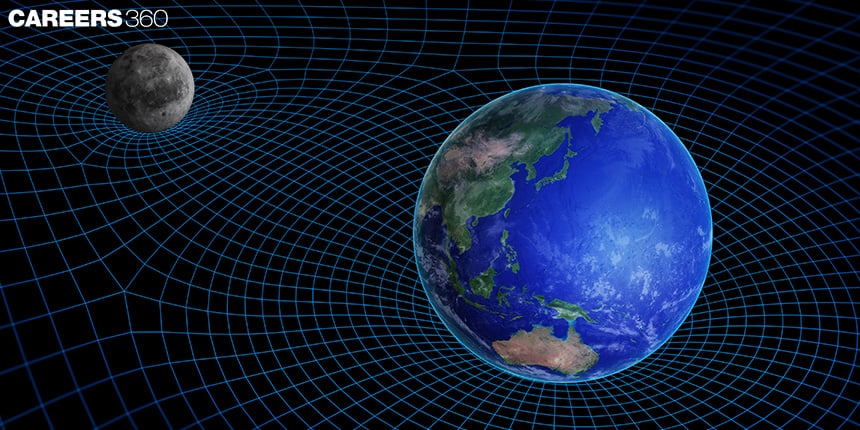###### Synopsis

The Law of Gravitation, proposed by Sir Isaac Newton, describes the gravitational force between two point masses proportional to their masses and inversely proportional to the distance between them. This article provides information on the value of g at different surfaces, myths and facts of gravitation, which is important for Class 11th students studying Physics. Understanding these concepts can enhance students' knowledge and prepare them for further studies in related subjects.###### Synopsis

The Law of Gravitation, proposed by Sir Isaac Newton, describes the gravitational force between two point masses proportional to their masses and inversely proportional to the distance between them. This article provides information on the value of g at different surfaces, myths and facts of gravitation, which is important for Class 11th students studying Physics. Understanding these concepts can enhance students' knowledge and prepare them for further studies in related subjects.

The law of gravitation, first proposed by Sir Isaac Newton in 1687, explains how matter attracts matter. It's a fundamental law that describes the force that holds everything together. It's the foundation of our understanding of the cosmos. The law of gravitation dictates the way objects interact with each other; it is the force that keeps planets in orbit around stars, stars in galaxies, and galaxies in clusters.

The law of gravitation acts as the primary law across the universe, shaping its structure and governing the motion of celestial bodies.

## Understanding The Force Of Attraction Between Celestial Bodies

According to Newton's Law of Gravitation, every point mass in the universe is attracted to every other point mass with a force that is proportional to the product of their masses and inversely proportional to the square of the distance between them. This indicates that the gravitational force between two objects is a function of their masses and their separation from one another, and it always acts to pull them together. This simple yet powerful law explains the behaviour of celestial bodies and the formation and evolution of celestial structures throughout the universe.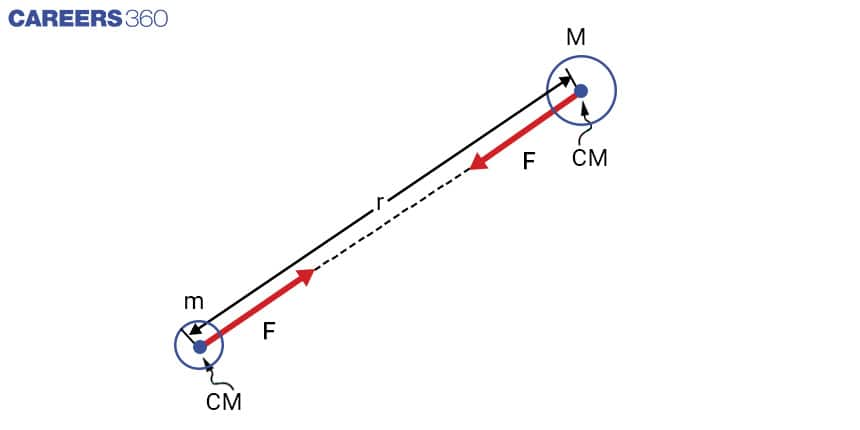In order to simplify the study of gravitational interactions between celestial bodies, we often act as if each object has all its mass concentrated at its centre of mass, like a single point. By treating each body in this way, we can use the Law of Gravitation to calculate the force of attraction between two bodies, represented by masses m and M, separated by a distance r.

In mathematical terms, the formula for the gravitational force is

where F is the force, The two objects' masses are m and M, and r is the distance between them. G is a gravitational constant that is considered to be constant throughout the universe. Experimental measurements have shown it to be

G = 6.674*10-11 Nm2kg-2

This simple formula has been tested and proven to be accurate in a wide range of situations, from the motion of planets in our solar system to the behaviour of galaxies in distant clusters.

## Understanding The Direction Of Gravitational Forces Between Bodies

One body's gravitational pull on another has a certain direction. So, a vectorised version of the above expression is possible. The gravitational forces in vector form for the bodies m and M separated by a distance r are as follows.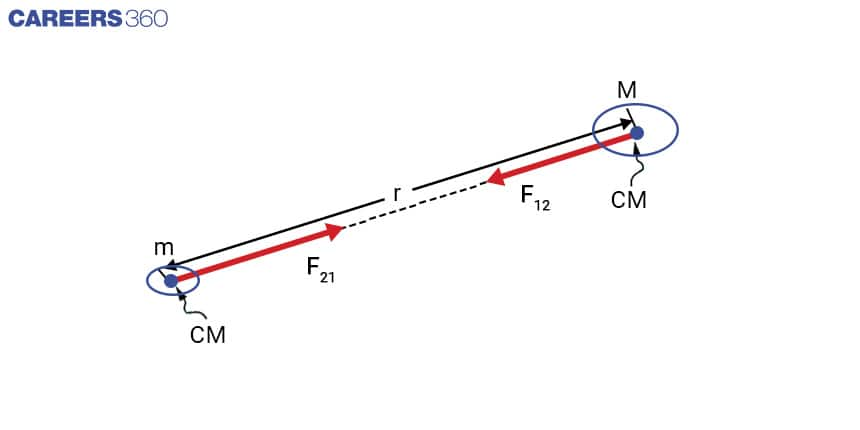The force F12 exerted on m by M is similarly to the force F21 exerted on M by m. The forces acting on both bodies are opposed in direction but have identical magnitude, with each body pulling the other towards it.

## Value Of g At Different Surface

The value of g, or the acceleration due to gravity, is generally considered to be constant and equal to 9.8 metres per second squared (m/s2) at sea level on Earth. However, this is an average value and it can vary slightly depending on location due to variations in Earth's density and shape.

Additionally, other factors such as altitude or height above sea level, latitude, and proximity to large masses such as mountains can also affect the value of g. At higher altitudes, the value of g is lesser due to the decrease in gravitational pull from the Earth. Conversely, at lower altitudes, such as in a mine, the value of g can be slightly more due to the increase in gravitational pull from the surrounding rock.

Also, the value of g is not the same on other celestial bodies, it varies from planet to planet, moon to moon and even on asteroids and comets.

### Value Of g On Earth's Surface

The value of g on the Earth's surface, also known as standard gravity, is given by the formula:

Where:

G = gravitational constant (approximately 6.674*10-11 Nm2kg-2)

M = mass of the Earth (approximately 5.972*1024 kg)

r = distance from the centre of the Earth to the point of measurement (approximately 6,371,000 meters for the Earth's mean radius)

By plugging in these values, we get:

gs = 6.674*10-11 Nm2kg-2 * (5.972 x 1024kg) / (6,371,000 m)2

gs = 9.8 m/s2 (approx)

Keep in mind that this is an average value of g at sea level and it can vary slightly depending on location due to variations in the Earth's density and shape.

### Value Of g At Height ‘h’ Above The Earth's Surface

To determine the value of g at point A, which is illustrated in the figure below, at a height h above the surface of the Earth. The value can then be determined as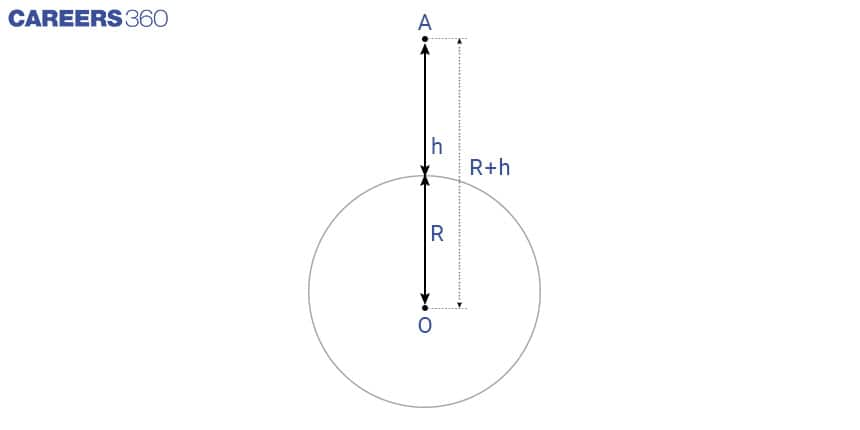In Case of point A is very close to Earth’s surface, then for h <<R and expression will be

### Value of g at a Depth h Below the Earth's Surface

The value of acceleration due to gravity (g) at a depth d below the Earth's surface can be calculated using the equation:

### gd= g (R – d)/R

Where

gd = Acceleration due to gravity at this depth is given by,

h < R

or

(1 – h/R) < 1

Therefore, gd < g

The acceleration due to gravity (g) decreases as one moves deeper towards the centre of the Earth. This change in g value is only noticeable when one moves very deep within the Earth. As a result, the value of g is not constant and varies with both height and depth.

## Myths And Facts

Myth: Newton discovered the law of gravity when an apple fell on his head.

Fact: While this story is often told, there is no evidence that it actually happened. Newton was inspired to study gravity after observing the fall of an apple, but it is unlikely that he was struck by one.

Myth: Gravity is a force that pulls objects towards the centre of the Earth.

Fact: Gravity is actually a force that attracts all objects with mass towards each other. This is why objects fall to the ground when dropped because the Earth's mass is pulling them towards it.

Myth: Gravity is the only force that affects objects in space.

Fact: While gravity is certainly a major force at play in space, there are many other forces at work as well. For example, objects in orbit around a planet are affected by the planet's gravity, but they are also affected by forces such as friction, air resistance, and electromagnetic forces.

Myth: Gravity is a mysterious force that scientists don't understand.

Fact: While there is still much to learn about gravity, scientists have a basic grasp of how it operates. It is generally acknowledged and well-tested that Newton's law of gravitation, which states that the force of gravity between two objects is inversely proportional to the square of their distance and proportionate to the product of their masses, is true.

Myth: Gravitational force acts only between objects on earth.

Fact: Gravitational force acts between all objects with mass, regardless of where they are in the universe. The force of gravity between the Earth and the Moon, for example, is what causes the tides on Earth, and the force of gravity between the Sun and the planets is what keeps the planets in their orbits.

• Science
• Nature
• Physical sciences
• Mass
• Physics
• Physical quantities
• Force
• Classical mechanics
• Mechanics
• Metrology

## Careers360 helping shape your Career for a better tomorrow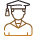#### 250M+

Students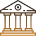#### 30,000+

Colleges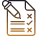#### 500+

Exams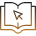E-Books